# Mathematics

Mathematics is an area of knowledge that includes the topics of numbers, formulas and related structures, shapes and the spaces in which they are contained, and quantities and their changes. These topics are represented in modern mathematics with the major subdisciplines of number theory, algebra, geometry, and analysis, respectively. There is no general consensus among mathematicians about a common definition for their academic discipline. Most mathematical activity involves the discovery of properties of abstract objects and the use of pure reason to prove them. These objects consist of either abstractions from nature or—in modern mathematics—entities that are stipulated with certain properties, called axioms. A proof consists of a succession of applications of deductive rules to already established results. These results include previously proved theorems, axioms, and—in case of abstraction from nature—some basic properties that are considered as true starting points of the theory under consideration. Mathematics is essential in the natural sciences, engineering, medicine, finance, computer science and the social sciences. The fundamental truths of mathematics are independent from any scientific experimentation, although mathematics is extensively used for modeling phenomena. Some areas of mathematics, such as statistics and game theory, are developed in close correlation with their applications and are often grouped under applied mathematics. Other mathematical areas are developed independently from any application (and are therefore called pure mathematics), but practical applications are often discovered later. A fitting example is the problem of integer factorization, which goes back to Euclid, but which had no practical application before its use in the RSA cryptosystem (for the security of computer networks). Historically, the concept of a proof and its associated mathematical rigour first appeared in Greek mathematics, most notably in Euclid's Elements. Since its beginning, mathematics was essentially divided into geometry and arithmetic (the manipulation of natural numbers and fractions), until the 16th and 17th centuries, when algebra and infinitesimal calculus were introduced as new areas of the subject. Since then, the interaction between mathematical innovations and scientific discoveries has led to a rapid lockstep increase in the development of both. At the end of the 19th century, the foundational crisis of mathematics led to the systematization of the axiomatic method. This gave rise to a dramatic increase in the number of mathematics areas and their fields of applications. This can be seen, for example, in the contemporary Mathematics Subject Classification, which lists more than 60 first-level areas of mathematics. (Wikipedia).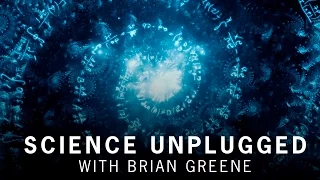What is mathematics?

From playlist Science Unplugged: MathematicsWhat is the point?

Putting an opinion on YouTube - what could possibly go wrong! This is a bit more bloggy than I usually like to do. I'll be back next time with some proper mathematics. "What is the point of mathematics?"

From playlist My Maths Videos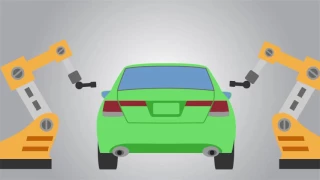Using Algebra and Geometry in the Real World

You hear terms like “algebra” and “geometry” and these theories we memorized in high school start to dance a jig in our heads – a jig many of us weren’t overly interested in! But the past decade has seen an explosion of applications of algebra, geometry, and topology to the real world, lik

From playlist What is math used for?The Impact of Mathematics on the World Around You

What can the STEM field do for humanity? How does the work professionals in the mathematical sciences are doing impact communities, countries, and the world? Applied math contributes to almost every aspect of our lives. Learn more from leading researchers and mathematicians who are making

From playlist What is math used for?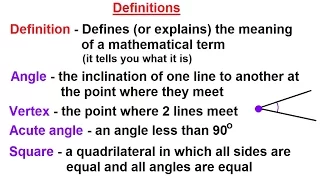Geometry: Ch 5 - Proofs in Geometry (2 of 58) Definitions

Visit http://ilectureonline.com for more math and science lectures! In this video I will explain and give examples of definitions. Next video in this series can be seen at: https://youtu.be/-Pmkhgec704

From playlist GEOMETRY 5 - PROOFS IN GEOMETRY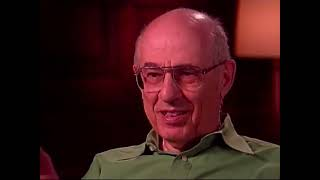Logic: The Structure of Reason

As a tool for characterizing rational thought, logic cuts across many philosophical disciplines and lies at the core of mathematics and computer science. Drawing on Aristotle’s Organon, Russell’s Principia Mathematica, and other central works, this program tracks the evolution of logic, be

From playlist Logic & Philosophy of Mathematics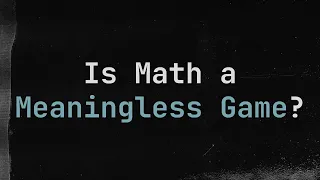What is Mathematics, Really? #SoME2

"What is mathematics?" and "What do mathematicians do?" Mathematics seems daunting or deeply nerdy. In my view, it's another way to look at the world, the same as art or science. Let's do some mathematics ourselves, speeding through the process from asking a question to telling others what

From playlist Summer of Math Exposition 2 videos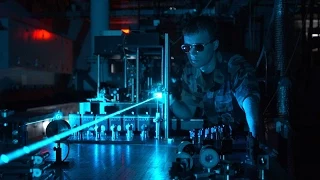Maths vs Science

What's the difference between maths and science? Free ebook http://tinyurl.com/VectorsYT

From playlist Math is Fun!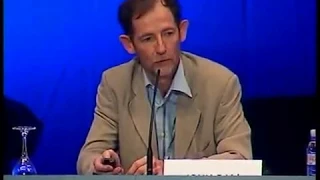ICM 2006 Round Table

ICM 2006 Closing Round Table Are pure and applied mathematics drifting apart? Intervention by John Ball (Slides https://www.mathunion.org/fileadmin/IMU/Videos/ICM2006/tars/table2006_ball.pdf) Intervention by Lennart Carleson (Slides https://www.mathunion.org/fileadmin/IMU/Videos/ICM2006/

From playlist Number Theory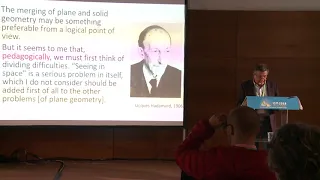On theories in mathematics education and their conceptual differences – Luis Radford – ICM2018

Mathematics Education and Popularization of Mathematics Invited Lecture 18.1 On theories in mathematics education and their conceptual differences Luis Radford Abstract: In this article I discuss some theories in mathematics education research. My goal is to highlight some of their diffe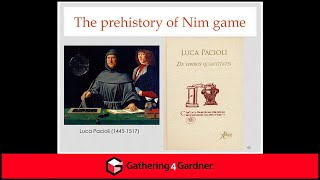Lisa Rougetet - The Role of Mathematical Recreations in the 17th and 19th Centuries - CoM Apr 2021

The aim of this talk is to retrace the history of mathematical recreations since the first books entirely dedicated to them at the beginning of the 17th century and at the end of the 19th century, especially in Europe. I will explain what mathematical recreations were exactly when they fir

From playlist Celebration of Mind 2021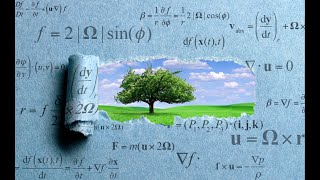Does Math Reveal Reality?

Mathematics has an uncanny ability to describe the physical world. It elegantly explains and predicts features of space, time, matter, energy, and gravity. But is this magnificent scientific articulation an invention of the human mind or is mathematics indelibly imprinted upon the substrat

From playlist WSF Latest Releases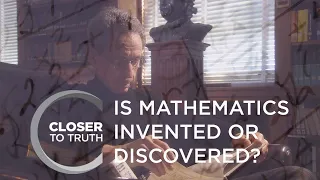Is Mathematics Invented or Discovered? | Episode 409 | Closer To Truth

Mathematics describes the real world of atoms and acorns, stars and stairs, with remarkable precision. So is mathematics invented by humans-like chisels and hammers and pieces of music? Or is mathematics discovered-always out there, somewhere, like mysterious islands waiting to be found? F

From playlist Closer To Truth | Season 4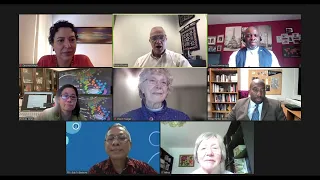CWM-CoD Joint Panel: Best practices towards a more diverse and inclusive mathematical community

Joint Panel organized by the IMU Committee for Women in Mathematics (CWM) and the IMU Committee on Diversity (CoD) CWM was established in 2015 to discuss issues related to women in mathematics worldwide and has actively worked to promote international contacts between national and region

From playlist IMU Live Events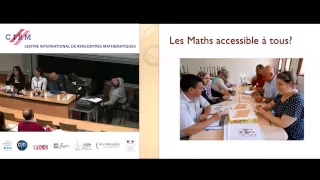Table ronde : mathématiques vivantes dans le monde

Find this video and other talks given by worldwide mathematicians on CIRM's Audiovisual Mathematics Library: http://library.cirm-math.fr. And discover all its functionalities: - Chapter markers and keywords to watch the parts of your choice in the video - Videos enriched with abstracts, b

From playlist Forum mathématiques vivantes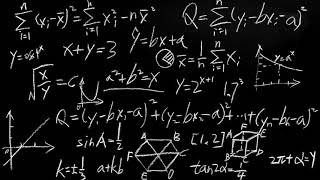What Are Numbers? Philosophy of Mathematics (Elucidations)

What is mathematics about and how do we acquire mathematical knowledge? Mathematics seems to be about numbers, but what exactly are numbers? Are numbers and other mathematical objects something discovered or invented? Daniel Sutherland discusses some of these issues in the philosophy of ma

From playlist Logic & Philosophy of Mathematics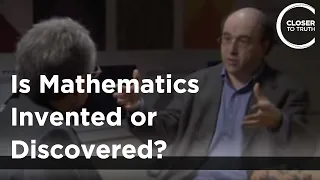Stephen Wolfram - Is Mathematics Invented or Discovered?

For more videos and information from Stephen Wolfram http://bit.ly/1GStsOr For more videos on whether mathematics is invented or discovered http://bit.ly/1DG70Hk To buy episodes and seasons of Closer To Truth click here http://bit.ly/1LUPlQS Mathematics describes the real world of atoms